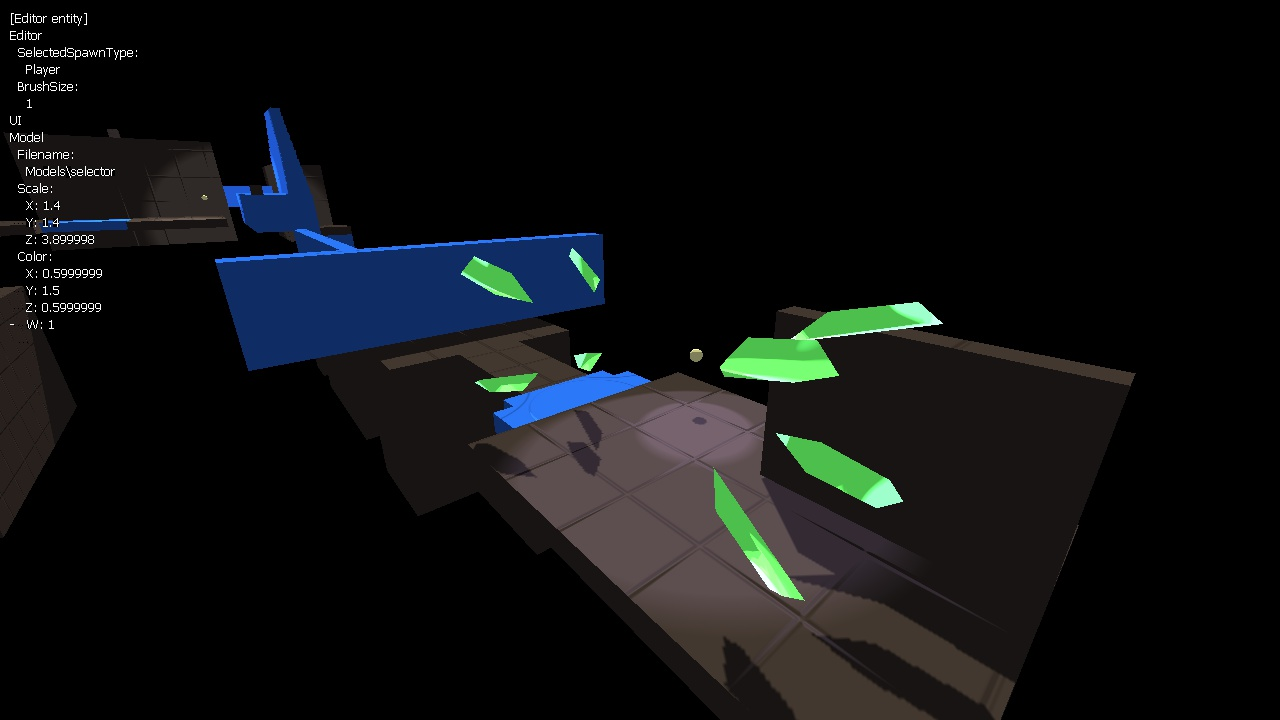# Back on track

I finished refactoring with components, added a reflection-enabled editor and XML serialization, finally got real PCF shadow filtering, and started experimenting with a somewhat cel-shaded visual style. I've been busy.

The refactoring had a lot of positive side effects. Serialization is now relatively easy, as the state of each component is determined by its properties, which are usually simple, easily serializable value types. One interesting side effect of serialization is that object creation is now split into two parts: initialization, and binding. When creating a new object at runtime, a factory performs the initialization. When loading objects from XML, the XmlSerializer performs initialization. In both cases, the factory creates the necessary bindings between properties afterward.

So far this works great on PC, but apparently XmlSerializer performance is significantly slower on Xbox. I'll report back as to whether this becomes an issue.

The new editor interface also uses reflection, making it extremely flexible at the cost of performance. Hopefully this won't be an issue either. Here's a screenshot.The interface is mostly keyboard (or gamepad) driven. When no entity is selected, it allows you to edit the properties of the editor itself. That way, I can make a property on the editor for the entity type to spawn, or the editor brush size, or any number of other useful editor thingies. Of course, this also means you can edit the scale and color of the editor model itself. It's quite amusing.I managed to reduce the controls down to two buttons plus movement, and I think I can add one more button without making it too complex. For Xbox, I'm thinking those will be the A button and the left and right triggers. On PC I'm thinking spacebar, Z, and X, with the arrow keys controlling movement. I'm still trying to nail down a few more moves and their control bindings. They'll probably change during play testing.

At any rate, as you can tell, the graphics have taken a step toward cartoon-land. For me, that means simpler textures and running all the lighting calculations through the following filter:

```float lightingFilter(float x)
{
float y;
float epsilon = 0.05f;
float a = 0.33333333f;
float b = 0.66666666f;
float c = 1.0f;
if(x < a - epsilon)
y = 0.0f;
else if(x < a)
y = (x - (a - epsilon)) * (a / epsilon);
else if(x < b - epsilon)
y = a;
else if(x < b)
y = a + (x - (b - epsilon)) * ((b - a) / epsilon);
else if(x < c - epsilon)
y = b;
else
y = b + (x - (c - epsilon)) * ((c - b) / epsilon);
return y;
}```

There are three pivot points (a, b, and c) that split the brightness spectrum into sections. Basically it makes the brightness ramp look like a step function instead of linear. At first I tried a straight up step function, but the transitions were a little too harsh, so I added small linear transitions between the levels. When the lighting value is within a certain epsilon value of one of the three pivot points, the output scales smoothly between the light levels. I'm sure you could do this with a polynomial and avoid all the branching, but I didn't want to think that hard.

I also finally implemented PCF filtering. Code for this is all over the internet, but I'll post my solution here just in case. It's nice and modular for easy plagiarism, at any rate.

```float GetShadowValue(float4 position, sampler2D shadowMap, float2 shadowMapSize)
{
// Get the shadow map depth value for this pixel
float depth = 1.0f - (position.z / position.w);

// Convert from clip space to UV coordinate space
float2 ShadowTexClipPosition = 0.5 * position.xy / position.w + float2(0.5f, 0.5f);

// Depth bias to prevent artifacts
float bias = 0.0005f;

// UV coordinates of the uppermost, leftmost pixel

// Collect samples from the surrounding four shadow map pixels
// These will all evaluate to 1.0 or 0.0
float bl = tex2D(shadowMap, pos).r - bias < depth; // Bottom left sample
float br = tex2D(shadowMap, pos + float2(1 / shadowMapSize.x, 0)).r - bias < depth; // Bottom right sample
float tl = tex2D(shadowMap, pos + float2(0, 1 / shadowMapSize.y)).r - bias < depth; // Top left sample
float tr = tex2D(shadowMap, pos + (1 / shadowMapSize)).r - bias < depth; // Top right sample

// Blend between the four samples
float horizontalBlend = (ShadowTexClipPosition.x - pos.x) * shadowMapSize.x;
float verticalBlend = (ShadowTexClipPosition.y - pos.y) * shadowMapSize.y;
float bottom = (bl * (1.0f - horizontalBlend)) + (br * horizontalBlend);
float top = (tl * (1.0f - horizontalBlend)) + (tr * horizontalBlend);
return ((bottom * (1.0f - verticalBlend)) + (top * verticalBlend)) * 10.0f;
}```

And here's the result:Notice the awkward light level transitions on that point light. Difficult cel-shading is difficult.